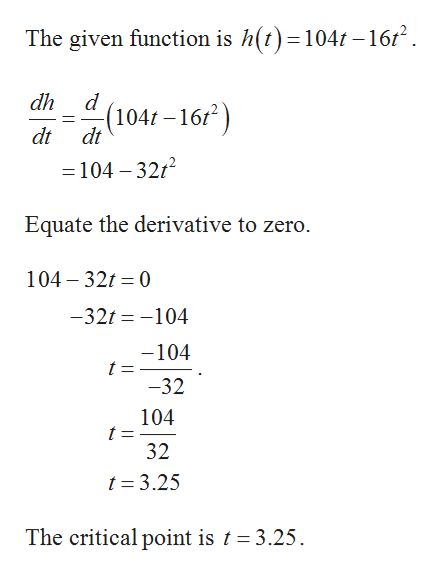# A ball is thrown vertically upward. After t seconds, its height h (in feet) is given by the function h(t)=104t - 16t2 . After how long will it reach its maximum height?Do not round your answer.

Question

A ball is thrown vertically upward. After t seconds, its height h (in feet) is given by the function h(t)=104t - 16t2 . After how long will it reach its maximum height?

check_circle

Step 1

In order to find the maxima or minima, first find the derivative and equate the derivative to zero, which is the critical point(s), then substitute the point(s) in second derivative to check the maxima and minima.

If the second derivative is less than zer...help_outlineImage TranscriptioncloseThe given function is h(t)=104t -1612 dh d (104r-16 dt dt =104 -3212 Equate the derivative to zero. 104 32 0 -32t-104 -104 t = -32 104 t = 32 t 3.25 The critical point is t 3.25 fullscreen

### Want to see the full answer?

See Solution

#### Want to see this answer and more?

Solutions are written by subject experts who are available 24/7. Questions are typically answered within 1 hour.*

See Solution
*Response times may vary by subject and question.
Tagged in

### Other